QSOs/candidates positioned onto galaxy disks, with notes. All photos are DSS, of 3 arcmin sides, and the QSO is seen at the precise center of each photo. If the text does not identify the precise location, the photo name is its J2000 co-ordinates. Note that QORG-calculated QSO likelihoods are too high for objects found within the isophotal galaxy disks, due to the presence of starbursts and APM/USNO-A optical artefacts. Such objects are included here on speculation.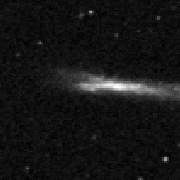NBCKDE004749.1-095401 (r=17.2, photometric QSO z=0.8) just under edge of edge-on galaxy MCG-2-3-16 (SBcd, z=0.0045).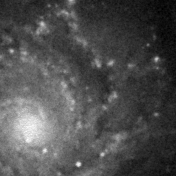CXOMP J013637.7+154740 (r=20.1, o=22.3, QSO prob=75%) is seen within disk of NGC 628 (Sc, z=0.0025).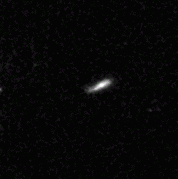QORG J013714.4-133856 (r=17.9, o=18.1, PSPC detected, QSO prob=84%) at the edge of MCG-2-5-21 (Sb).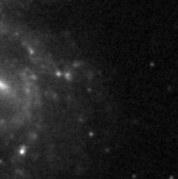QSO NGC1073U1 (r=19.7, o=19.4, z=1.941) seen within NGC 1073 (SBc, z=0.0040)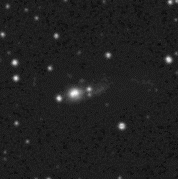QSO S4 0248+43 (r=15.7, o=17.6, z=1.31) on disrupted limb of double galaxy IRAS 02483+4302 (Sc, z=0.0516).QORG J033334.2-361103 (r=18.6, bj=19.8, PSPC detected, QSO prob=61%) next to where the arm joins the body of NGC 1365 (SBb, z=0.0051).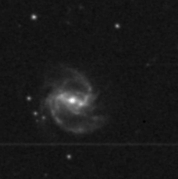QORG J044147.1-011756 (r=17.5, o=17.1, RASS detected, QSO prob=73%) positioned on the arm of UGC 3134 (SBc, z=0.029). Not a QSO, see 2008A&A,480,61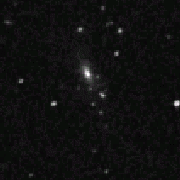QORG J045720.0-313902 (r=18.0, bj=18.4, RASS detected, QSO prob=69%) at the end of ESO 422-14 (Sbc).QORG J051319.4-414302 (r=18.3, bj=18.1, PSPC detected, QSO prob=65%) at the end of FAIR 1133 (Sa, z=0.0807).QORG J070916.8+203747 (r=18.5, o=18.1, RASS detected, QSO prob=75%) on the arm of NGC 2342 (Sc, Z=0.017).SDSS J075402.8+363936 (QSO, r=18.1, o=17.6, z=0.586) next to the end of galaxy KUG 0750+367 (Sc).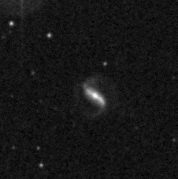QORG 081517.4+460437 (r=17.3, o=16.2, QSO prob=69%) at the base of the arm(s) of MCG 8-15-56 (Sc, z=0.041).NBCKDE082014.3+441346 (r=19.8, photometric QSO z=0.3) on the halo of CGCG 207-56 (z=0.024).NBCKDE083432.6+001141 (r=16.8, o=18.1, photometric QSO z=0.3) on the rim of barred spiral CGCG 4-60 (z=0.037).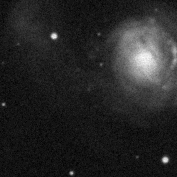CXOMP J091410.2+400617 (r=19.9, o=20.3, QSO prob=89%) on outer region of NGC 2782 (SBa, z=0.0085).NBCKDE101100.4+443430 (r=20.1, photometric QSO z=0.4) on edge of KUG 1007+448 (Sc, z=0.0283).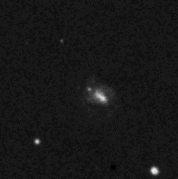NBCKDE101922.1+490710 (r=19.2, photometric QSO z=0.5) at disrupted end of MCG 8-19-19 (Sb, z=0.0544).SDSS J102114.0+565959 (QSO, r=19.7, z=1.053) at lower edge of colliding galxies MCG 10-15-67 (SBb, z=0.0098).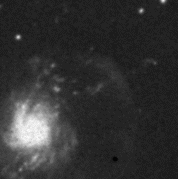CXOMP J103839.7+533053 (r=18.7, QSO prob=67%) on linear ejection from NGC 3310 (SBbc, z=0.003).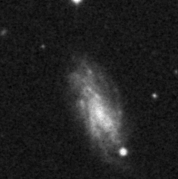NBCKDE103937.5+472416 (r=19.4, photometric QSO z=0.8) is positioned well within NGC 3320 (SBc, z=0.008).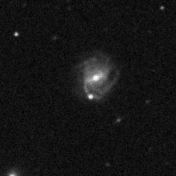QORG J104439.8+384525 (r=16.6, o=16.9, QSO prob=80%) shines thru the arm of MCG 7-22-53 (Sbc, z=0.035).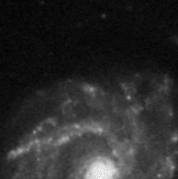CXOMP J112103.7+531139 (r=17.1, o=17.1, QSO prob=60%) on the disk of NGC 3631 (Sc, z=0.004).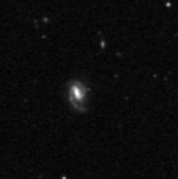NBCKDE112735.9+002346 (r=20.3, photometric QSO z=1.0) at the edge of CGCG 11-100 (Sb, z=0.0491).SDSS J114010.1+455724 (QSO, r=18.4, z=0.506) on the outside regions of UGC 6628 (SBm, z=0.003).NBCKDE115503.5+582942 (r=19.7, photometric QSO z=1.0) on disk of NGC 3963 (SBbc, 0.0106).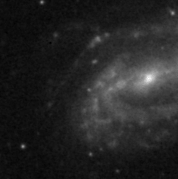NBCKDE120815.1+025229 (r=19.2, photometric QSO z=1.4) positioned on arm of NGC 4123 (SBc, z=0.0045).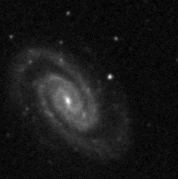NBCKDE121230.9+120736 (r=21.0, photometric QSO z=0.3) positioned on arm of IC 769 (SBbc, z=0.0073).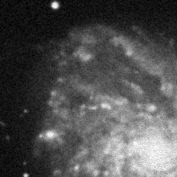QORG J121854.2+142606 (r=19.0, o=19.7, QSO prob=73%, RASS detected) is seen on disk of NGC 4254 (Sc, z=0.0079).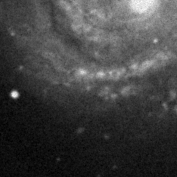QORG J122258.7+154751 (r=19.5, o=21.1, QSO prob=91%, HRI detected) is seen on disk of NGC 4321 (SBbc, z=0.0050).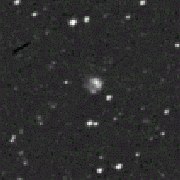QSO PKS 1221-42 (r=17.9, bj=17.9, z=0.171) at the bottom of galaxy 6dF J122343.3-423531 (z=0.0266).QORG J124346.1+162327 (r=19.6, o=20.9, QSO prob=57%, PSPC detected) on an arm of NGC 4651 (Sc, z=0.0030).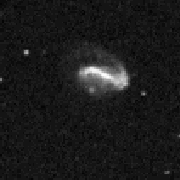QORG J124615.7-132156 (r=18.9, o=19.1, QSO prob=72%, NVSS detected) at bottom of MCG-2-33-6 (SBcd, z=0.017).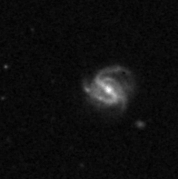NBCKDE124848.3+415515 (r=16.0, o=15.8, photometric QSO z=0.8) on the leftward arm of NGC 4704 (SBbc, z=0.027).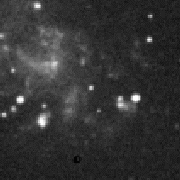NBCKDE130428.7-033444 (r=18.8, o=18.9, photometric QSO z=0.5) on galaxy MCG 0-33-28 (SBd, z=0.0046).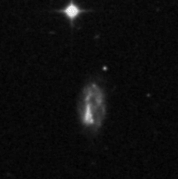QSO BG 57 5 (r=17.4, o=18.2, z=0.79) at north end of galaxy MCG 5-31-133 (SMm, 0.016).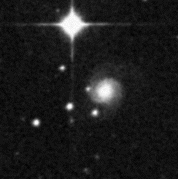QSO MS 13173-1213 (r=15.0, o=16.3, z=0.329) on the edge of MCG-2-34-31 (Sc, z=0.010).NBCKDE132259.6+525807 (r=20.7, photometric QSO z=0.3) to lower right of center of galaxy MCG 9-22-54 (Sb, z=0.0305).NBCKDE132979.9+582548 (r=19.6, o=20.2, photometric QSO z=0.7) on outer reaches of NGC 5204 (Sm, z=0.001).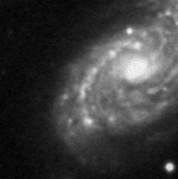NBCKDE133735.2+085247 (r=19.1, photometric QSO z=1.3) on inside of arm of NGC 5248 (SBbc, z=0.0038).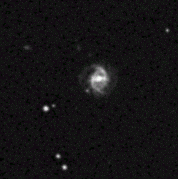NBCKDE133914.9+441451 (r=18.1, o=18.5, photometric QSO z=2.0) off rump of barred spiral CGCG 218-32 (z=0.034).NBCKDE140828.8+070341 (r=20.8, photometric QSO z=1.3) just north of centre of UGC 9037 (Sc, z=0.0198).NBCKDE141026.6+591528 (r=17.9, o=19.6, photometric QSO z=0.3) on edge of MCG 10-20-81 (E-SO, z=0.0426).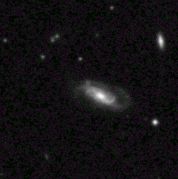QORG J142618.6+261452 (r=17.0, o=17.2, QSO prob=77%, PSPC detected) on north arm of IC 4423 (Sbc, z=0.030).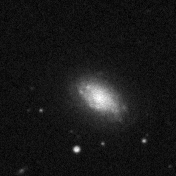NBCKDE143026.4+351926 (r=19.0, photometric QSO z=0.9) in disk of NGC 5656 (Sab, z=0.011).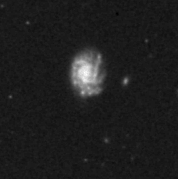NBCKDE143542.6+081747 (r=16.2, o=15.7, photometric QSO z=1.0), just below center of disk of NGC 5681 (Sb, z=0.026).NBCKDE153448.4+283923 (r=19.4, photometric QSO z=1.1) on disk (at 1 o'clock) of NGC 5958 (Sc, z=0.007).NBCKDE154601.7+024251 (r=20.8, photometric QSO z=1.3) just to left of center of CGCG 50-95 (z=0.0126).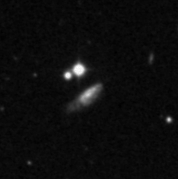NBCKDE155200.2+424343 (r=20.8, photometric QSO z=1.9) in disk (upper right of center) of MCG 7-33-3 (Sc, 0.0345).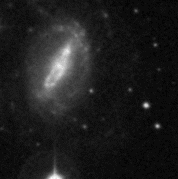QORG J155411.8+143540 (r=18.0, o=18.8, QSO prob=45%, RASS detected) on outer part of NGC 6012 (SBab, z=0.006).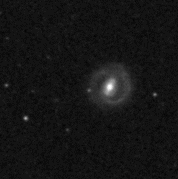NBCKDE160145.0+484937 (r=19.5, o=20.2, photometric QSO z=0.3) on arm of MCG 8-29-33 (z=0.0429).QORG J161301.4+371710 (r=15.5, o=15.7, RASS detected, QSO prob=79%) is buried in arm at 5o'clock from nucleus of MCG 6-36-3 (Sc, z=0.07).SDSS J163317.7+352018 (QSO, r=18.5, z=0.231) is just at the south tip of the bright nucleus of NGC 6185 (Sa, z=0.034).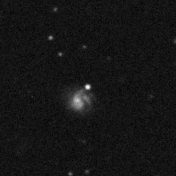NBCKDE164001.3+434151 (r=16.9, o=17.4, photometric QSO z=1.1) outshines MCG 7-34-129 (Sb, z=0.0349).NBCKDE 171015.7+283452 (r=19.3, photometric QSO z=0.5) is positioned on a bend in the arm of MCG 5-40-46 (Sc, z=0.045).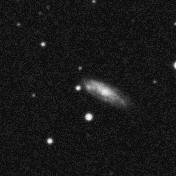NBCKDE171611.9+314736 (r=19.9, photometric QSO z=1.3) is within the left edge of UGC 10781 (Sbc, z=0.0229).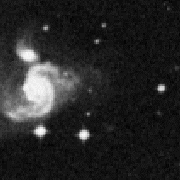QORG 220903.7-274835 (r=19.3, o=20.4, QSO prob=79%, HRI detected) lies in outfall of disrupted galaxy NGC 7214 (SBbc, z=0.0227).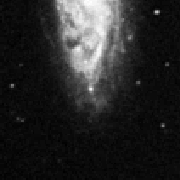1XMM J223546.3-260429 (r=17.6, o=18.6, QSO prob=69%) lies on south disk of NGC 7314 (SBbc, z=0.0049).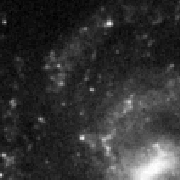CXOMP J225723.8-410255 (r=18.8, bj=19.3, QSO prob=95%) in disk of NGC 7424 (SBc, z=0.0031).

Notes:

(1) NBCKDE photometric quasars are from the DR3 catalog provided on their site.

(2) QORG objects are from the QORG catalog (2004 A&A 427, 387). Note that RASS-detected objects may not be (in my subjective opinion) as reliable as HRI / PSPC-detected objects.

(3) 1XMM and CXOMP detections are X-ray only. For presentation on this page, these detections were associated to optical objects, and the probability of each associated optical object being a QSO calculated, using the data-driven QORG algorithm.

If using these in published research, please cite this site.